## "If you can't explain it simply, you don't understand it well enough" (Albert Einstein)## Uso del módulo de corte “G” en análisis de asentamientos de cimentaciones bajo cargas estáticas

Traditionally, in Geotechnical Engineering, the deformation modulus E has been used for the estimation of settlements in foundations under static loads. However, if the mechanical behavior of the soil is considered, the use of the shear modulus G is more convenient, even though it is not frequently employed for this type of analysis. Do you want to learn more about this topic? Continue reading this post...

### Mechanical behavior of a material

In order to understand the use of the shear modulus G in the estimation of settlements under static loads, we will first take a look at the mechanical behavior of a material, in order to recognize certain basic concepts.

For a given material, the relationship between stresses and strains is governed, as is logical to suppose, by its properties. Thus, in the presence of an ideally rigid material, strains would be zero and movements could only occur if there were a mechanism that generates them. For real materials, it happens that they can be compressed (or stretched) or deformed, as shown in Figure 1, taken from the text of Atkinson (2007).

Part (a) of the figure shows a block of material subjected to a confining pressure σ and part (c) shows a relationship between this pressure and the change in volume, the gradient of which is the volumetric modulus K. As can be seen from this graph, the stress can be increased more or less indefinitely, and the material will continue to compress stably without reaching failure, while the modulus K continues to increase with stress and strain.

On the other hand, part (b) of Figure 1 shows a block of material subjected to shear stress τ, which causes it to deform upon shearing. Part (d) illustrates a simple relationship between shear stress and shear strain, whereby the gradient is the shear modulus G, which decreases with stress and strain. Thus, the material fails when no more shear stress can be added and continues to deform at a constant shear stress τf, which represents the shear strength of the material.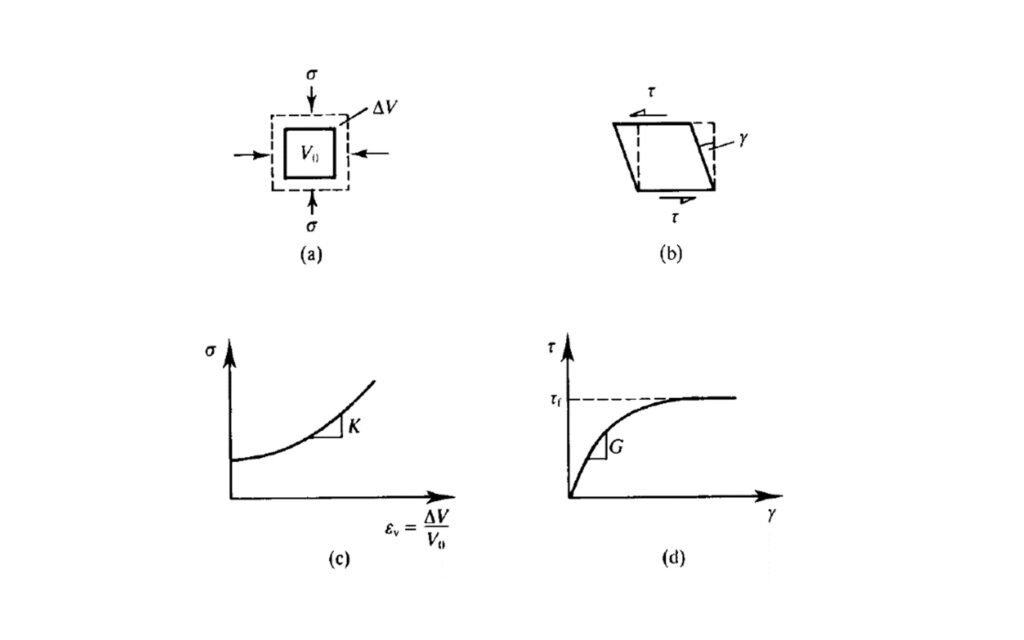Figure 1 Compression and distortion of a material (Source: Atkinson, 2007).

As can be seen from the figure we are analyzing, the compression observed in part (a) implies a change in size, while the shear distortion of part (b) implies a change in shape.

In general, in any material (and thus also in soil) under load, compression and distortion occur simultaneously. That is why the stiffness of the material relates the changes in stresses and changes in strains by means of the following expressions, based on the graphs in Figure 1: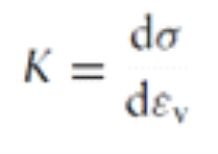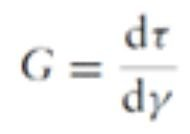where εv = V/Vo is the volumetric strain and γ is the shear strain. The simplest theory of stiffness is the Theory of Elasticity, where K and G are constants and apply equally to loading and unloading.

### Selection of soil stiffness parameters

In Geotechnical Engineering, the selection of soil stiffness parameters depends on both the drainage conditions (i.e., whether or not the soil could undergo volume changes) and the level of strains (Simons & Menzies, 2000).

In the case of Young's modulus E, when considered under drained or effective conditions (typically denoted as E'), this parameter incorporates both volumetric and shear strains. However, when considered under undrained or total stress conditions (denoted as Eu), it only incorporates shear strains, without considering volume changes.

In this context, the use of Young's modulus as a soil stiffness parameter is attractive from a practical point of view, mainly because it is a relatively easy concept to understand from a stress-strain curve; and because it can be obtained from relatively simple laboratory tests (although not necessarily with an adequate level of accuracy with respect to in situ conditions).

However, based on the mechanical concepts reviewed in the previous section, a better approach to the problem of establishing soil stiffness includes the use of the volumetric modulus K and the shear modulus G, which would allow separating soil deformation into two components: volumetric (involving a change in size without shear deformation), and distortional (associated with a change in shape at constant volume).

An extremely important fact to note, is that the shear modulus G is independent of drainage conditions, which means that, in terms of numerical analysis, when the loading conditions change from an undrained to a drained state, G remains constant, while E must adapt from Eu to E' (Simons & Menzies, 2000).

The typical variation of shear modulus G with strains for most soils is shown in Figure 2. According to this figure, it is evident that the soil has a non-linear behavior, as explained in a previous post. (see https://geo-webonline.com/que-significa-que-el-suelo-tiene-un-comportamiento-no-lineal/).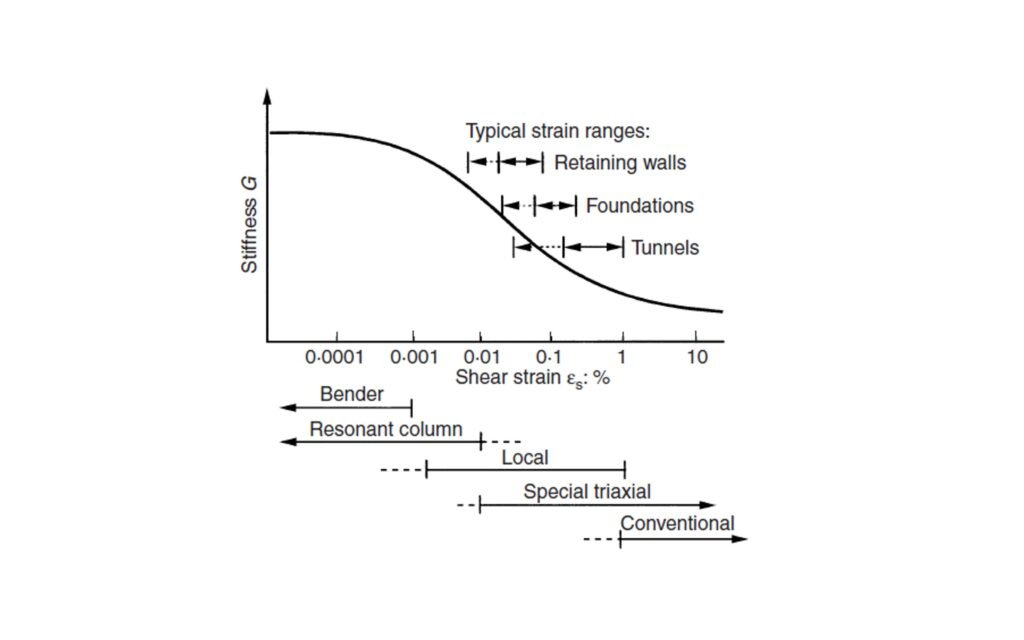Figure 2 Variation of stiffness with strains (Source: Simons & Menzies, 2000).

There are numerous practical methodologies for constructing the stiffness curve of a given soil, some of which are even standardized (see, for example, EUROCODE 7). Usually, the value of Go corresponding to very small strains is determined experimentally, and from there the rest of the curve. An important detail is that Go can be determined from in situ geophysical tests (such as down-hole, for instance), which results in an enormous cost-benefit advantage for the project.

Returning to the figure, it can be seen that a typical characteristic ground deformation for foundations is about 0.1%, a value that is equivalent to a foundation settlement of the order of 10 mm in a 10 m deep compressible profile (Atkinson, 2007).

Thus, knowing the variation curve of G vs γ, it is possible to estimate values of G corresponding to the expected strains for a given structure, and subsequently estimate the value of E' (typically required in the available models for settlement estimation) from the following expression, proposed by the Theory of Elasticity: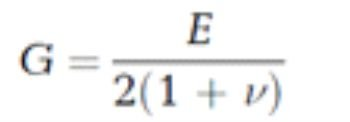However, a very common question arises in practice: how to determine the value of Eu if it were necessary to estimate, for example, short-term settlement in clays? Again, on the basis of what is established by the Theory of Elasticity. As pointed out by Lambe & Whitman (1969) in their classic work on Soil Mechanics, the relationship between the deformation modulus E for different drainage conditions is given by:

Eu = 1,15

Regarding this ratio, the aforementioned authors warn that for particularly soft soils, with high deformation potential, this ratio can reach values of 3 or 4, which implies that settlements would be governed by long-term deformation, as indeed occurs in soils susceptible to consolidation.

### Why use G-modulus in foundation settlement analysis?

To answer this question, it is first necessary to recognize that there is a sort of barrier in many Geotechnical Engineering professionals, who consider that, as has historically been the case, the G-module is valid only for dynamic analyses. However, it is only necessary to take a look at the previous sections, or at the high level of knowledge we have reached on the subject over the last 50 years (at this point, I consider that the references of Jardine et al, 1985; Atkinson, 2000; Oztoprack & Bolton, 2013; and Vardanega & Bolton, 2013; Cox & Mayne, 2015 - which I leave you at the end of the post - can be of great use to go deeper into the subject), to understand that this is not the case.

In fact, the use of the G-modulus in settlement estimations (following the process described briefly in section 2 of this post) has the advantage that the deformation moduli obtained are closer to the in situ deformation moduli. This can be seen in Figure 3 below.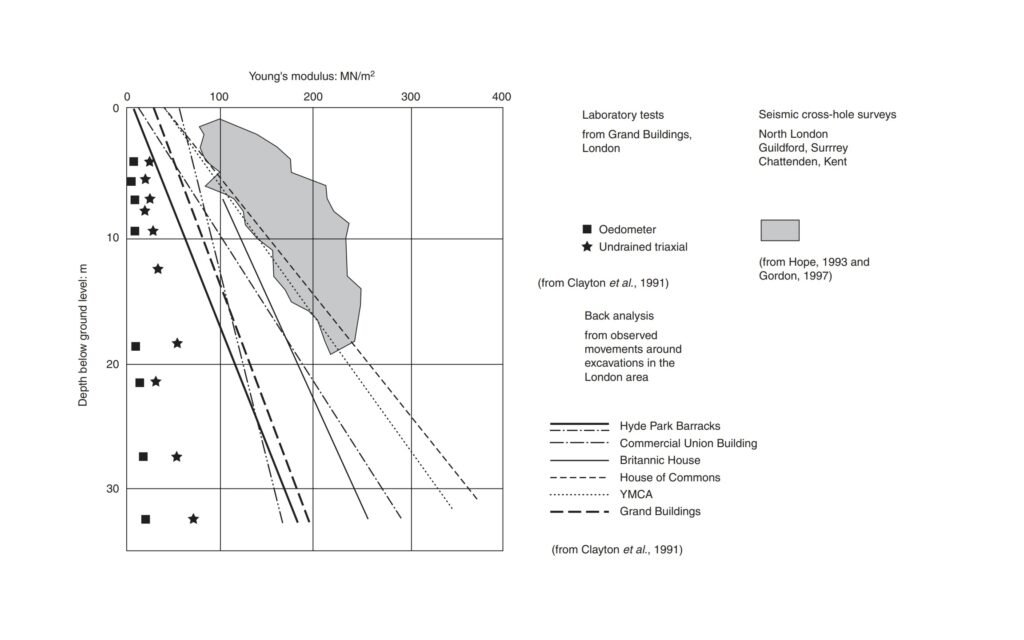Figure 3 Stiffness profiles for several grounds located in London, UK (Source: modified from Simons & Menzies, 1999).

In this graph, we clearly see the relationship between Eu deformation moduli obtained from: 1) conventional laboratory tests; 2) back-analysis from structure motion measurements; and 3) geophysical tests.

Thus, based on what is illustrated in this figure, I consider it appropriate to highlight two fundamental facts:

• Laboratory tests significantly underestimate the values of modulus of deformation with respect to in situ conditions.

• Geophysical tests allow obtaining the value of Go, from which it is possible to estimate G for each particular analysis.

According to the above, it is evident that the use of the shear modulus G as a fundamental parameter for the estimation of foundation settlements under static loads provides a better approximation to the real estimation of soil stiffness, which, in turn, allows optimizing the foundation design.

### References

• Atkinson, J. (2007) “The Mechanics of Soils and Foundations”. Second Edition. CRC Press – Taylor & Francis Group. Abingdon, UK.
• Atkinson, J. H. (2000) “No-lineal Soil Stiffness in Routine Design”. Geotechnique 50, No. 5, 487-508.
• Cox, C. & Mayne, P. (2015) “Soil Stiffness Constitutive Model Parameters for Geotechnical Problems: A Dilatometer Testing Approach”. Third Conference on DMT, no. 12. Rome, Italy.
• Jardine, R.; Fourie, A.; Maswoswe, J. & Burland, J. (1985) “Field and Laboratory Measurements of Soil Stiffness”. Proceedings of the Eleventh International Conference on Soil Mechanics and Foundation Engineering, pp. 511-514. San Francisco, USA.
• Mitchell, J.K. & Soga, K. (2005) “Fundamentals of Soil Behavior”. Third Edition. John Wiley & Sons, Inc. New Jersey, USA.
• Oztoprack, S. & Bolton, M. (2013) “Stiffness of Sands through a Laboratory Test Database”. Geotechnique 63, No. 1, 54–70 (http://dx.doi.org/10.1680/geot.10.P.078).
• Simons, N. & Menzies, B. (2000) “A Short Course in Foundations Engineering”. 2nd. Edition. Thomas Teldford Limited 2000. London, UK.
• Vardanega, P. & Bolton, M. (2013) “Stiffness of Clays and Silts: Normalizing Shear Modulus and Shear Strain”. Journal of Geotechnical and Geoenvironmental Engineering, Vol. 139, No. 9. ©ASCE, ISSN 1090-0241/2013/9-1575–1589.